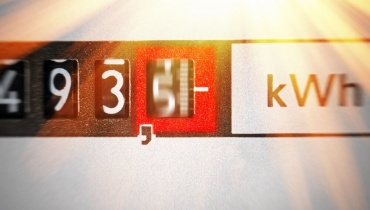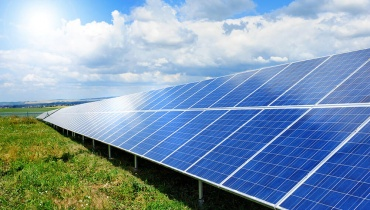# In a FlashA watt hour measures the amount of energy used over a period of time.

## kW and kWh explained

Kilowatts (kW) and kilowatt hours (kWh) are units used to measure energy. They're based on watts (W), which measures rates of power (the rate at which energy is produced or consumed) in a period of time.

# Burning Questions

## What is a watt?

Let's start with the basics! A watt (W) is a unit of power, and power is the rate at which energy is produced or consumed. A watt measures rates of power over a time period.

You could think of watts as a measure of electrical flow. Picture an electrical device - does it need a big flow or a small flow to work? Here's what we mean:

• A brighter light bulb (a 100 W bulb) uses energy at a higher rate than a dimmer light bulb (a 60 W bulb).
• This means the brighter light bulb needs a bigger electrical flow to work - that's why it has a higher wattage.

It's the same with solar energy - the rate at which your solar energy system ‘flows' the power into your school is measured in watts.

## So what's a kilowatt?

A kilowatt just means 1000 watts - simple!

## What is a watt-hour?

All the electrical appliances and devices in your home - from your fridge to your TV - need energy in the form of electricity in order to work.

A watt-hour (Wh) is a unit used to measure the amount of this electrical energy used over time.

1 Wh = 1 W of power expended for 1 hour of timeCompact fluorescent (CFL) and light-emitting diode (LED) bulbs provide just as much light as incandescent lightbulbs, and they consume less energy.

## And what's a kilowatt hour?

One kilowatt hour (kWh) means one kilowatt of power transferred or consumed in one hour.

1 kWh = 1 kW of power expended for 1 hour of time

As you may have guessed, a kilowatt hour is equal to 1000 watt-hours. You usually pay for the energy you use by the kilowatt hour.

## How is solar energy measured?

Solar energy is measured in kilowatt hours - or with large solar energy systems, in megawatt hours (1000 kilowatt hours).

## Solar energy measurement in action:

If your solar panels continuously output 1 kW of power for a period of 1 hour, they'll have produced 1 kWh of energy.

# What Do You Mean?How much are your electrical devices are costing you?

Power is the rate at which energy is produced or consumed.

Watts (W) measure rates of power over a period of time.

A kilowatt (kW) is 1000 watts.

A watt-hour (Wh) is a unit that measures the amount of electrical energy used over a period of time.

A kilowatt hour (kWh) is 1000 watt-hours.

A megawatt hour (mWh) is 1000 kilowatt hours.

# Cool Facts

If you leave your TV or computer on ‘standby', it's still using power - this means it's adding a kWh cost to your energy bill!

# Cool Facts

Watts are named after the Scottish inventor James Watt, who also invented the steam engine.

# Speedy SummarySolar energy, is measured in kilo-Watt-hours (kWh) or with large solar installations, mega-Watt-hours (mWh)

A watt (W) measures the rate at which energy is produced or consumed. 1000 watts is called a kilowatt (kW). We usually pay for our electrical energy based on the amount of kilowatt hours (kWh) used - this is the equivalent to 1 kW of power expended over 1 hour of time.

# Teacher's Toolkit

Take this to the classroom!